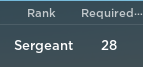# Rank not changing once reached goal, Custom Rank System

Hey, I was making a non-group ranking system for a game and im having a problem on changing the rank after reaching the RequiredToRank stat:

So what I want to achieve is once the players RequiredToRank stat reaches the required amount to get a new rank, it should change the Rank stat to the next rank but totally not working

Stats Script:

``````local datastore = game:GetService('DataStoreService'):GetDataStore('RS12345')

local stat = Instance.new('Folder')
stat.Parent = p

local key1 = 'rank'
local key2 = 'required'

local rank = Instance.new('StringValue')
rank.Name = 'Rank'
rank.Parent = stat
rank.Value = datastore:GetAsync(key1) or 'Recruit'

local required = Instance.new('IntValue')
required.Name = 'RequiredToRank'
required.Parent = stat
required.Value = datastore:GetAsync(key2) or 0

required.Value += 1
end
end)
``````

RankUp Script:

``````local ranks = {
 = {Rank = 'Private'},
 = {Rank = 'Private First Class'},
 = {Rank = 'Corporal'},
 = {Rank = 'Sergeant'},
 = {Rank = 'Staff Sergeant'},
 = {Rank = 'Sergeant First Class'},
 = {Rank = 'Master Sergeant'},
 = {Rank = 'First Sergeant'},
 = {Rank = 'Sergeant Major'},
 = {Rank = 'Command Sergeant Major'},
 = {Rank = 'Second Lieutenant'},
 = {Rank = 'Lieutenant'}
}

local datastore = game:GetService('DataStoreService'):GetDataStore('RS12345')

local rank = stat.Rank
local require = stat:WaitForChild('RequiredToRank')

for required,nextrank in pairs(ranks) do
if require.Value >= required then
rank.Value = nextrank.Rank
end

if require.Value <= required and rank.Value == nextrank.Rank then
rank.Value = nextrank.Rank
end
end
end)

game.Players.PlayerRemoving:Connect(function(p)

local key1 = 'rank'
local key2 = 'required'

if rank and required then
datastore:SetAsync(key1,rank.Value)
datastore:SetAsync(key2,required.Value)
end
end)
``````

That is because you’re only checking the player’s rank when he joins, not when his requirement changes.

Inside the .PlayerAdded event, put this:

``````local require = stat:WaitForChild('RequiredToRank')

require.Changed:Connect(function()
for required,nextrank in pairs(ranks) do
if require.Value >= required then
rank.Value = nextrank.Rank
end

if require.Value <= required and rank.Value == nextrank.Rank then
rank.Value = nextrank.Rank
end
end
end)
``````

It should check your rank everytime your requirement changes. Let me know if you’re encountering any issue with that.

Have a good rest of your day.

2 Likes

Hey, thanks for replying, IT did change the rank but the rank gets stuck at some point…Are you sure the text is not truncated? Try setting the rank higher than 30

Not sure if this would work:

``````require:GetPropertyChangedSignal("Value"):Connect(function()
for required, nextrank in pairs(ranks) do
if nextrank[required] ~= nil then
rank.Value = nextrank.Rank
end
end)
``````

Make sure to close the `for` loop with “`end`

1 Like

Thanks, i didnt notice that.

30303030

Did some little changes:

``````local ranks = {
{Required = 1, Rank = 'Private'},
{Required = 3, Rank = 'Private First Class'},
{Required = 5, Rank = 'Corporal'},
{Required = 10, Rank = 'Sergeant'},
{Required = 15, Rank = 'Staff Sergeant'},
{Required = 25, Rank = 'Sergeant First Class'},
{Required = 30, Rank = 'Master Sergeant'},
{Required = 35, Rank = 'First Sergeant'},
{Required = 45, Rank = 'Sergeant Major'},
{Required = 60, Rank = 'Command Sergeant Major'},
{Required = 80, Rank = 'Second Lieutenant'},
{Required = 100, Rank = 'Lieutenant'}
}
``````
``````	for _,nextrank in pairs(ranks) do
if require.Value >= nextrank.Required then
``````

and surprisingly did not give me any problems.

This topic was automatically closed 14 days after the last reply. New replies are no longer allowed.